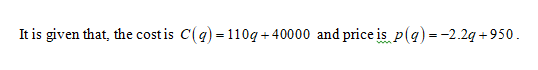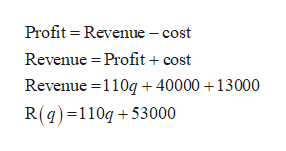# Given Cost and Price​ (demand) functions C(q)=110q+40000 and p(q)=-2.2q+950, what is the marginal revenue when profits are​$13000? Question Asked Dec 19, 2019 11 views Given Cost and Price​ (demand) functions C(q)=110q+40000 and p(q)=-2.2q+950, what is the marginal revenue when profits are ​$13000?

check_circle

Step 1Step 2

Obtain the marginal revenue when profit is \$13000.help_outlineImage TranscriptioncloseProfit = Revenue – cost Revenue = Profit + cost Revenue =110g + 40000 +13000 R(q) =110g + 53000 fullscreen
Step 3

Differentiate the revenue to obt...

### Want to see the full answer?

See Solution

#### Want to see this answer and more?

Solutions are written by subject experts who are available 24/7. Questions are typically answered within 1 hour.*

See Solution
*Response times may vary by subject and question.
Tagged in

### Math